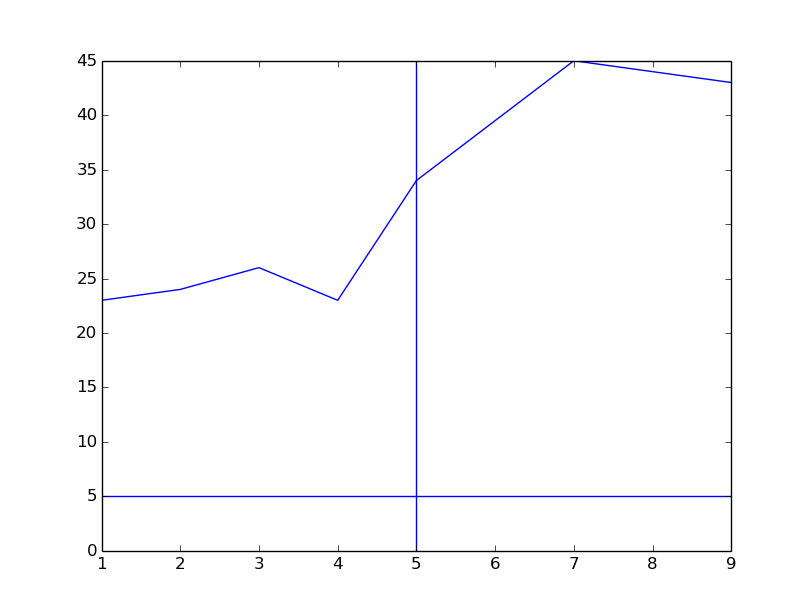## Plotting horizontal lines

Date: February 28th 2016
Last updated: February 28th 2016

Plotting horizontal (and vertical) lines can be done using axhline and axvline functions.

``````import matplotlib.pyplot as plt

# Create data
x = [1, 2, 3, 4, 5, 7, 9]
y = [23, 24, 26, 23, 34, 45, 43]

# plot
plt.plot(x, y)
plt.axhline(5)
plt.axvline(5)
plt.show()
``````